# 1. Graph the short-run total product curves for each of the following production functions if K...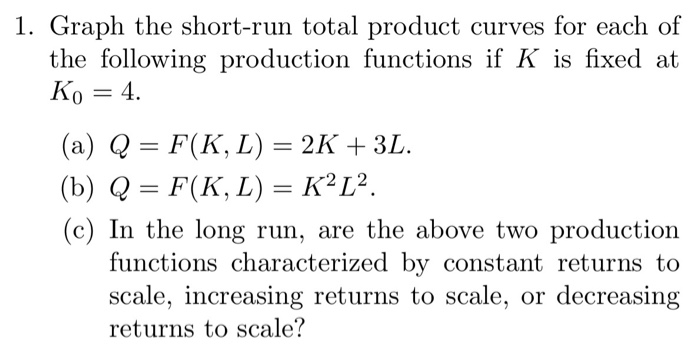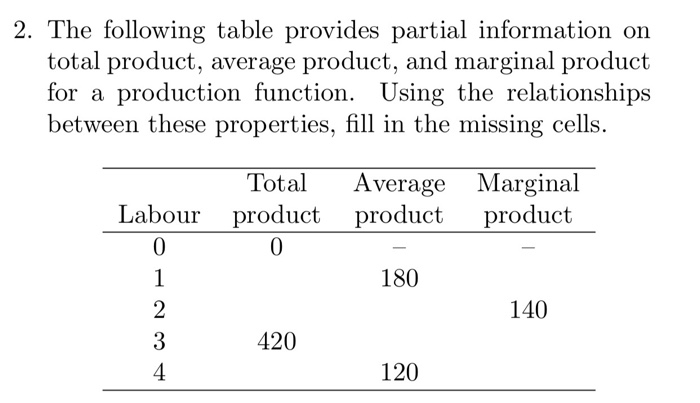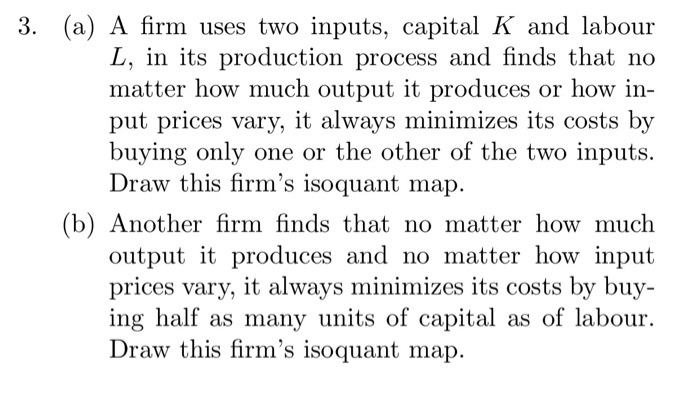1. Graph the short-run total product curves for each of the following production functions if K is fixed at Ko 4 (a) Q = F(K, L) = 2K + 3L. (b) Q = F(K, L) = K2L2. (c) In the long run, are the above two production functions characterized by constant returns to scale, increasing returns to scale, or decreasing returns to scale?

This Homework Help Question: "1. Graph the short-run total product curves for each of the following production functions if K..." No answers yet.

We need 10 more requests to produce the answer to this homework help question. Share with your friends to get the answer faster!

0 /10 have requested the answer to this homework help question.

Once 10 people have made a request, the answer to this question will be available in 1-2 days.
All students who have requested the answer will be notified once they are available.

#### Earn Coin

Coins can be redeemed for fabulous gifts.

Similar Homework Help Questions
• ### 1. A production function is given by f(K, L) = L/2+ v K. Given this form,...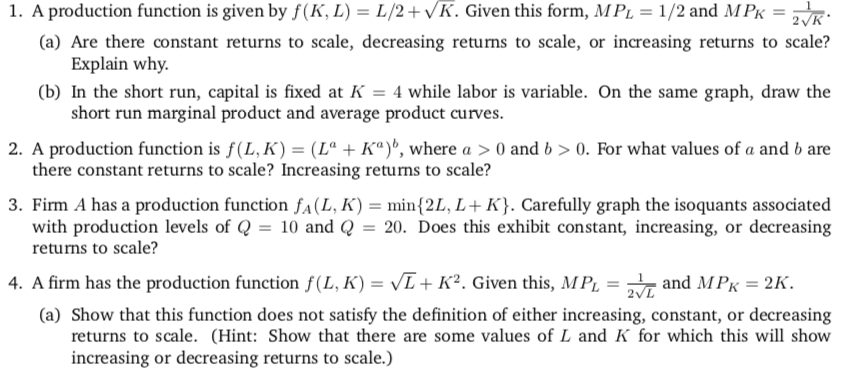1. A production function is given by f(K, L) = L/2+ v K. Given this form, MPL = 1/2 and MPK-2 K (a) Are there constant returns to scale, decreasing returns to scale, or increasing returns to scale? (b) In the short run, capital is fixed at -4 while labor is variable. On the same graph, draw the 2. A production function is f(LK)-(L" + Ka)", where a > 0 and b > 0, For what values of a and...

• ### 1. Below are production functions that turn capital (K) and labor (L) into output. For cach of th...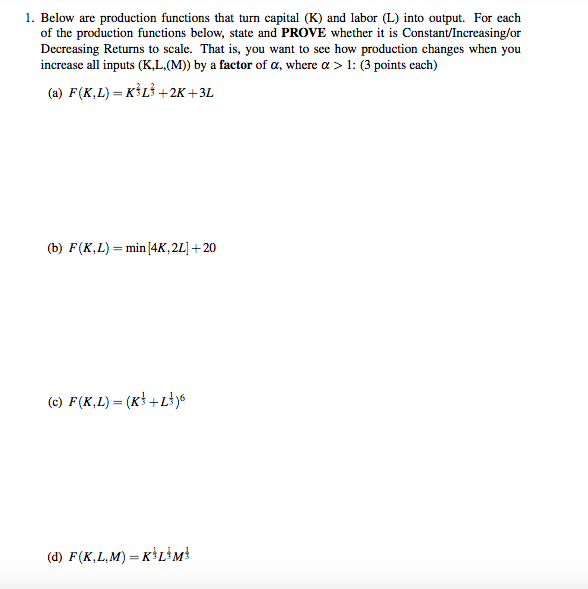1. Below are production functions that turn capital (K) and labor (L) into output. For cach of the production functions below, state and PROVE whether it is Constant/Increasing/or Decreasing Returns to scale. That is, you want to see how production changes when you increase all inputs (K,L, (M)) by a factor of a, where a > 1: (3 points each) (a) F(K,L)-KİLİ+2K +3L (b) F(K, L)=min/4K, 2L1+20 (d) F(K,L,M) KL3M 1. Below are production functions that turn capital (K) and...

• ### 5. Determine whether each of the following production functions displays constant, increasing, or decreasing returns to...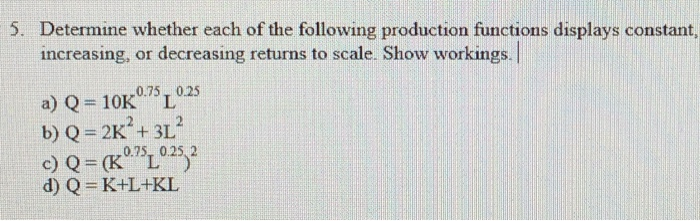5. Determine whether each of the following production functions displays constant, increasing, or decreasing returns to scale. Show workings. a) Q= 10K 0.75, 0.25 b) Q = 2K+ + 3L c) Q = (Kº75 0.25 2 d) Q=K+L+KL

• ### Short Run Cost Curves: Consider two firms, producing different products, with the following production functions: q=5KL...

Short Run Cost Curves: Consider two firms, producing different products, with the following production functions: q=5KL (1) q=5(KL).5 (2) a. For a short-run situation in which K=100, and given wage = 3 and cost of capital = 1, derive expressions short run total cost for each production function. (Start by using the production function to develop an expression for L in terms of q, and then substitute that, and the given parameters, into the generic expression for Total Cost =...

• ### 3. For each of the following production functions, graph a typical isoquant and determine whether the marginal rate of...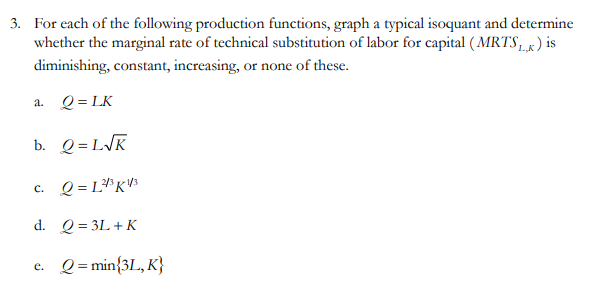3. For each of the following production functions, graph a typical isoquant and determine whether the marginal rate of technical substitution of labor for capital (MRTS ) is diminishing, constant, increasing, or none of these. a. Q=LK b. Q=LVK c. Q=L*K13 d. Q = 3L +K e. Q = min{3L, K} Show transcribed image text 3. For each of the following production functions, graph a typical isoquant and determine whether the marginal rate of technical substitution of labor for capital...

• ### 1. For the following production functions, find the marginal rate of technical substitu- tion (MRTSLK). Does the produc...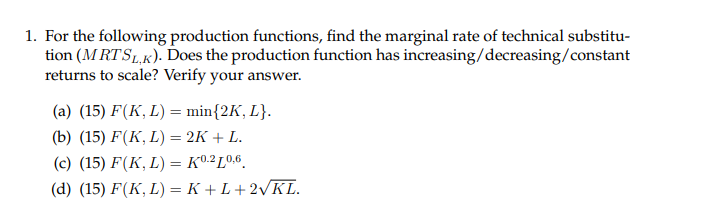1. For the following production functions, find the marginal rate of technical substitu- tion (MRTSLK). Does the production function has increasing/decreasing/constant returns to scale? Verify your answer (a) (15) F(K, L) = min{2K, L}. (b) (15) F(К, L) — 2K + L. (c) (15) F(K, L) = K0.2L0,6. (d) (15) F(K, L) — К +L+2VKL.

• ### Below are production functions that turn capital (K) and labor (L) into output. For each of...

Below are production functions that turn capital (K) and labor (L) into output. For each of the production functions below, state and PROVE whether it is Constant/Increasing/or Decreasing Returns to scale. That is, you want to see how production changes when you increase all inputs (K,L,(M)) by a factor of α, where α > 1: (4 points each) a) F(K,L) = K^1/3*L^1/3+2K+3 b) F(K,L) = sqr(K^3+L^3) c) F(K,L) = (K^2/4+L^2/4)^2 d) F(K,L,M) = min(K,L)*M

• ### The following graph shows short-run marginal cost curves, short-run average cost curves, and a long-run average...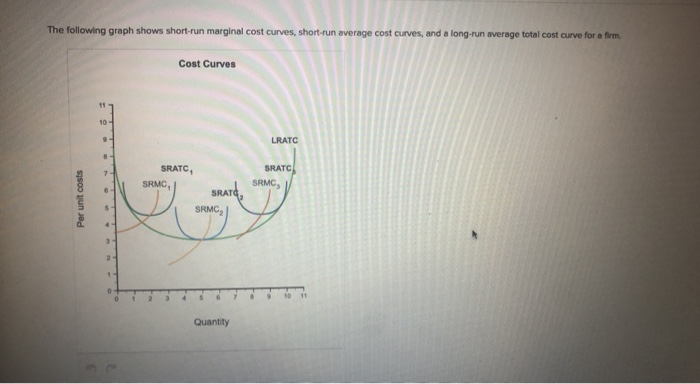The following graph shows short-run marginal cost curves, short-run average cost curves, and a long-run average total cost curve for a firm. Cost Curves 11 10 - 9 LRATC SRATC SRMC SRATC SRMC Per unit costs SRATO SRMC . 10 10 Quantity Which cost curves represent an efficient firm producing where there are diseconomies of scale? (Click to select) | Which cost curves represent an efficient firm producing where there are economies of scale? (Click to select) Which cost curves...

• ### 1. State whether the following production functions exhibit increasing, constant, or decreasing returns to scale in...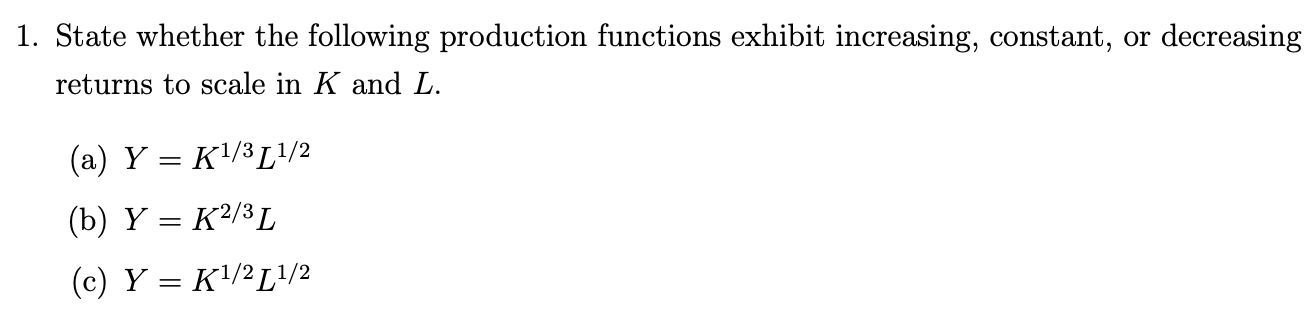1. State whether the following production functions exhibit increasing, constant, or decreasing returns to scale in K and L. (a) Y = K1/3L1/2 (b) Y = K2/3L (c) Y = K1/2 [1/2

• ### Question 2: Technologies -[22 TOTAL POINTS] Three different production functions can be represented mathematically as follows:...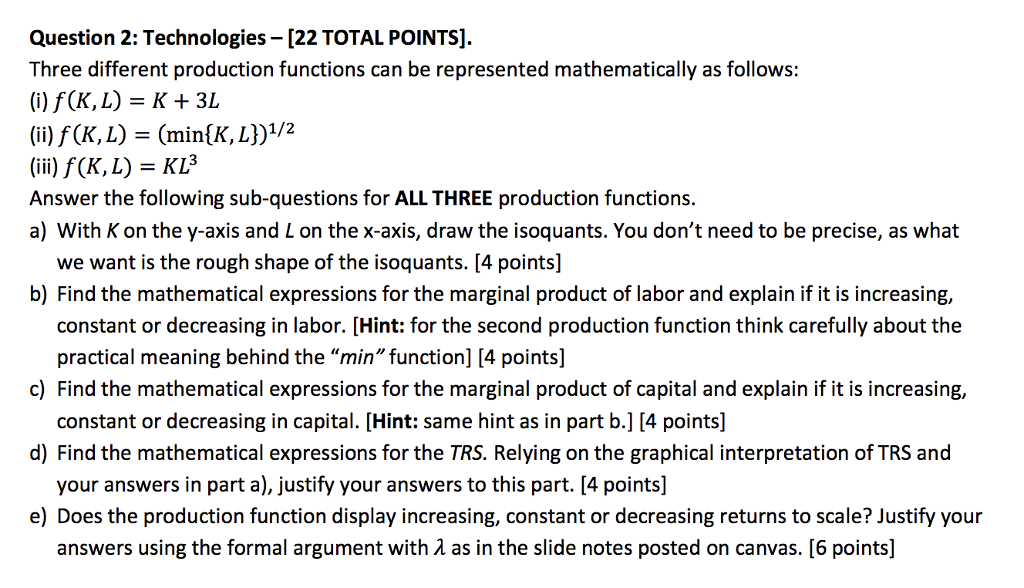Question 2: Technologies -[22 TOTAL POINTS] Three different production functions can be represented mathematically as follows: (i) f (K,L)K+3L (K,L) - (minlK, (iii) f (K, L) = K Answer the following sub-questions for ALL THREE production functions. a) with K on the y-axis and L on the x-axis, draw the isoquants. You don't need to be precise, as what we want is the rough shape of the isoquants. [4 points] b) Find the mathematical expressions for the marginal product of...

Free Homework App